﻿ Similar Constructing Method for Solving the Boundary Value Problem of the Compound Kummer Equation

### Similar Constructing Method for Solving the Boundary Value Problem of the Compound Kummer Equation

Duo Zhang, Shunchu Li, Dongdong GuiOPEN ACCESSPEER-REVIEWED

## Similar Constructing Method for Solving the Boundary Value Problem of the Compound Kummer Equation

Duo Zhang1, Shunchu Li1,, Dongdong Gui2

1College of Science, Xihua University, Chengdu, China

2Beijing Dongrunke Petroleum Technology Co., Ltd., Beijing, China

### Abstract

This article is devoted to analyze the compound Kummer equation. Through the structure of left region and right region similarity kernel function, have similar structure of solution for this boundary value problems. The left field solution of this kind of boundary value problems can be obtained by combing the coefficients of boundary value problems on the left and the left area similar kernel function. The right field solution of this kind of boundary value problems can be expressed by assembling the left, the right area similar kernel function, the left lead solution functions and the coefficients of the convergence conditions. Thus put forward the simple method which was named similar constructive method to solve the boundary value problems of the compound Kummer equation. The put forward of this method provides the convenience for solving this kind of differential equation boundary value problems.

### At a glance: Figures

12
Prev Next

• Zhang, Duo, Shunchu Li, and Dongdong Gui. "Similar Constructing Method for Solving the Boundary Value Problem of the Compound Kummer Equation." American Journal of Mathematical Analysis 3.2 (2015): 39-43.
• Zhang, D. , Li, S. , & Gui, D. (2015). Similar Constructing Method for Solving the Boundary Value Problem of the Compound Kummer Equation. American Journal of Mathematical Analysis, 3(2), 39-43.
• Zhang, Duo, Shunchu Li, and Dongdong Gui. "Similar Constructing Method for Solving the Boundary Value Problem of the Compound Kummer Equation." American Journal of Mathematical Analysis 3, no. 2 (2015): 39-43.

 Import into BibTeX Import into EndNote Import into RefMan Import into RefWorks

### 1. Introduction

The boundary value problems of differential equations always are needed in the calculation of many physical and engineering [1, 2, 3, 4, 5]. So it is not just the initial value problems of differential equation, boundary value problems are also the focus of our study. Compound boundary value problem of Kummer equation is a mathematical problem that closely associated with the practical application. But when in solution of the compound boundary value problem of Kummer equation, we often encounter the complicated solving processe. So it is inconvenience for the practical application. Some boundary value problems of differential equations can be written in the form of solution of continued fractions which was named similar structure type. And this conclusion in 2004 has been proved [6, 7, 8, 9, 10]. For example, the boundary value problem of Kummer equation, the boundary value problem of Euler hypergeometric equation. Then the solution of the boundary value problem for composite Kummer equations whether it has the above properties?

This article will study the following compound confluence hypergeometric equation boundary value problem:(1)

Whereare all known real constant, and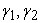are not as integers,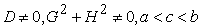.

### 2. Preliminary Knowledge

lemma 1  The general solution for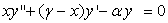is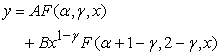(2)

Where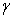is not an integer, both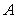and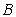are real constants and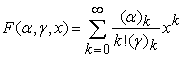lemma 2 Introducing two binary functions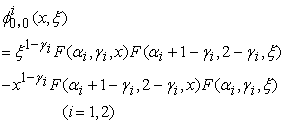(3)

then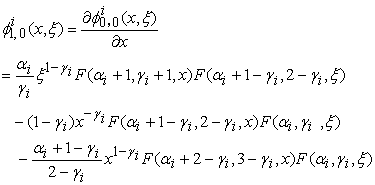(4)(5)(6)

Proof: Based on the differential properties of the Kummer function we can know that(7)

so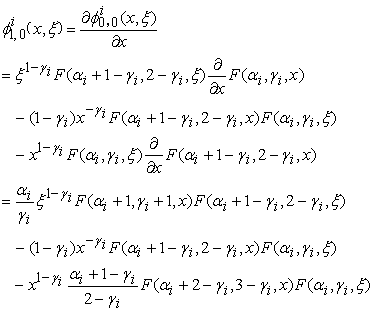Thus: Similarly verifiable (5) and(6).

### 3. The Main Theorem and Its Proof

Theorem If boundary value problem (1) has a unique solution, the left area () is:(8)

The right area (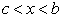) solution is:(9)

Whereis defined as similar kernel function of the right area :(10)

Whereis defined as similar kernel function of the left area :(11)

Proof: First of all, Based on the lemma 1 we know that the general solution for(12)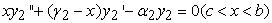(13)

are as follows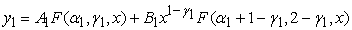(14)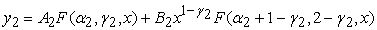(15)

Where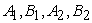are real constants.

According the left boundary conditions, we can know that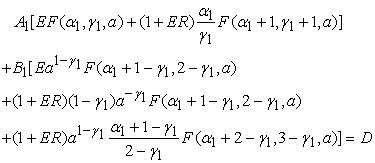(16)

According to the continuity conditions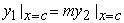,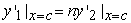we can get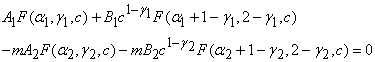(17)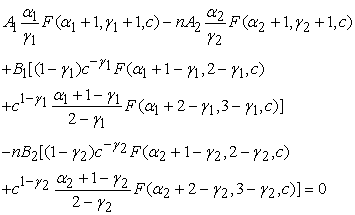(18)

By the right boundary conditions of the complex confluence hypergeometric equation boundary value problem (1) that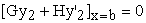we can get(19)

Getting linear equations about of undetermined coefficientsand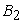by combining equation (16), (17), (18) and (19).

If we assume that complex confluence hypergeometric equation boundary value problem (1) has a unique solution, then there will be a determinant of coefficient of linear equations not be 0,mean that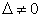.And after calculation using (3), (4), (5), (6) for simplifying we can get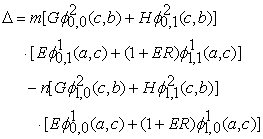(20)

Using Kramer rule to solve the equations set which is composed of (14), (15), (16) and (17) equations, we can get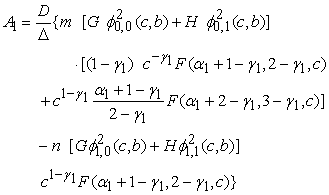(21)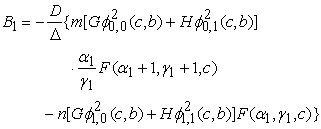(22)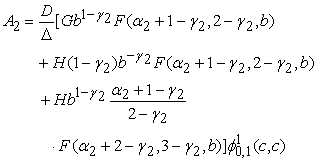(23)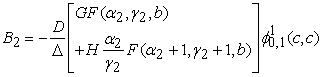(24)

Thencan be obtained if we getsubstitution of (14), (15). And if we simplifyusing the similar kernel function of the left and the right area, we can get the solution of the left and the right area (8) and (9), respectively.

### 4. Give an Example

Draw the image of following boundary value problem solution: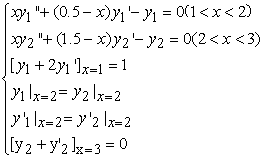(25)

According to the preliminary knowledge we can get the general solution of the target equation as follows.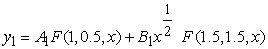(26)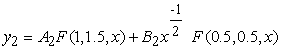(27)

Through the comparison of the toundary value problem (1) and the boundary value problem(25) we found that, using MATHEMATICA language program to draw the solution of the boundary value problem in the specified image definition domain as follows:

Figure 1. Cuver of solution to boundary value problem(25) (left area)

By changing the parameters of D and G in the condition of observation and analysis of the image change.

### 5. Conclusions and Understanding

1) With the definition of the left, the right area of similar kernel function (10) and (11), the solutions of boundary value problem (1) can be written as the continued fraction structure. In the progress of solving the boundary value problem for composite Kummer equation, we can get the left area and the right area solution directly by constructing similarity kernel function. This simplifies the solving process, improves the solution efficiency, contributes to the researchers compile corresponding analysis software.

2) From the Figure 3 and Figure 4 we can see that the greater absolute value of the parameter D the greater function curve fluctuations in the left area and the right area.

3) From the Figure 4 we can see that the image function on the X axis symmetry when D were obtained from the two opposite number.

4) From the Figure 5 we can see that the greater absolute value of the parameter G the greater function curve fluctuations in the left area.

5) From the Figure 5 we can see that in the right area regardless of how to change the parameters of the G image through a fixed point and the point coordinates for (2.71,-0.04748).

### Acknowledgment

This work is supported the by the Scientific Research Fund of the Sichuan Provincial Education Department of China (Grant No. 12ZA164).

### References

  Gaoxiong-Wang, Zhiming-Zhou, Siming-Zhu, Shousong-Wang. Ordinary Differential Equation[M]. Beijing:Higher Education Press, 1978.In article  Li Shun-chu. The Similarity Structuring Method of Boundary Value Problems of the Composite Differential Equations (in Chinese) [J]. Journal of Xihua University (Natural Science Edition), 2013, 32(4): 27-31.In article  Li Shun-chu, Wu Xiao-qing. Several Important Properties for the Boundary Value Problems of Second-order Linear Homogeneous Differential Equation (in Chinese)[J]. Journal of Xihua University (Natural Science Edition), 2013, 32(1): 23-26.In article  LI Shun-Chu, Liao Zhi-Jian. Constructing the Solution of Boundary Value Problem of the Differential Equation with it’s an Arbitrary Non-trivial Solution (in Chinese) [J]. Journal of Sichuan University (Natural Science Edition), 2012, 49(6): 1209-1213.In article  WANG Fu-rong, LI Shun-chu, XU Dong-xu. The Similarity Construction Method of a Kind of Boundary-Value Problem of Airy Equation(in Chinese) [J]. Journal of Hubei Normal University (Natural Science), 2013, 33(1):79-85.In article  Bao Xitao, Li Shunchu, Xiao Xuxia, Xu Dongxu. Similar Constructive Method of Solutions of Nonlinear Seepage Model for Homogeneous Reservoir (in Chinese)[J]. NATURAL GAS AND OIL, 2012, 30(65): 47-51.In article  LI Wei, LI Xiaoping, LI Shunchu, LI Quanyong. SIMILAR STRUCTURE OF THE SOLUTIONS OF MATHEMATICAL MODEL FOR THE NONLINEAR FLOW OF FRACTAL COMMINGLED OIL RESERVOIRS (in Chinese) [J]. Petroleum Geology & Oilfield Development in Daqing, 2012, 31(6): 79-83.In article  WANG Jun-chao, LI Shun-chu, XU Li. Spherical Fluids Flow Models in Composite Reservoir Based on Similar Structure of Solution (in Chinese)[J]. Journal of Guilin University of Technology, 2012, 32(4): 624-627.In article  LI Quan-yong, LI Shun-chu, LI Wei, WANG Jun-chao. Study of the Nonlinear Fluid Flow Model in Dual-porosity Media Reservoir Based on Similar Structure[J].CHINESE JOURNAL OF ENGINEERING MATHEMATICS,2013, 30(1):123-130.In article  LI Quan-yong, LI Shun-chu, LI Wei, WANG Jun-chao. Solution to Base on the Similar Structure of the Double Porosity-Multilayer Reservoir(in Chinese) [J]. College Mathematics, 2013, 29(1): 56-60.In article  LI Shun-chu, Huang Bing-guang. Laplace transform and Bessel functions and the theoretical basis of well test analysis [M].Beijing: Petroleum Industry Press, 2000.In article  LI Shun-chu,WANG Jun-chao,XU Li. The Similar Structure of Solutions to the Spherical Fluids Flow Problems for a Composite Reservoir (in Chinese)[J]. Journal of Mathematics in Practice and Theory, 2014,44(3):122-127.In article  Li Ke, Li Shunchu, Dong Yanan. The Solution of Well Test Analysis Model Based on Similar Structure in the Multilayer Reservoir (in Chinese)[J]. Well Testing, 2013, 22(2):7-9.In article  Xiao Xuxia, Li Shunchu. The Similar Structure of Solutions for the Boundary Value Problem of Euler’s Hypergeometric Differential Equation (in Chinese)[J]. Journal of Inner Mongolia Normal University (Natural Science Edition), 2012, 41(6): 597-600(-603).In article  BAO Xi-tao, LI Shun-chu, LIAO Zhi-Jian. Similar Constructive Method of Solution for the Boundary Value Problem of Euler Hyper-geometric Differential Equation (in Chinese)[J]. Journal of Southwest University of Science and Technology, 2012, 27(4):101-105.In article